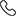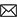Sales :9214233303 / Tech. Support Desk :9214233360/40 (9AM ~ 6PM)# CBSE Linear Programming & Probability for Class 12th (NCERT) by MC Sir

• Probability is a measure of the likelihood of an event. Higher probability events are more likely to occur than events with low probability. Mathematically, probability lies between 0 and 1, where 1 denotes the certainity of occurence of an event. It is a widely used mathematical concept, with applications ranging from computer science to finance.
• In this chapter, we shall discuss the important concept of conditional probability of an event given that another event has occurred, which will be helpful in understanding the Bayes' theorem, multiplication rule of probability and independence of events, also we shall study some linear programming problems and their solutions by graphical method only, though there are many other methods also to solve such problems.
• Rs.2,000 Rs.2,000
• Rs.2,000 Rs.2,000
• Rs.3,000 Rs.3,000
Subject Medium Target Language Mathematics English XII Class English + Hindi Topic Based 18 Videos 0 Videos PDF Files Full coverage of topics Linear Programming & Probability 12th class(N.C.E.R.T.) from the latest CBSE syllabus All questions fully solved of (Linear Programming & Probability)Exemplar Exercise Properly organized solutions in chapter-wise and page wise format help find your solution quickly Step-by-step model solutions to all question Helpful aid during exam preparation Class 11th students who are willing to go an extra mile to study Class 12th concepts in 11th itself. Class 12th students who are keen to pursue a course which covers 12th standard mathematics comprehensively and enables them to fulfill their dream of getting into IITs Essential for CBSE, NIOS & ICSE Exam preparations

### Other Courses by Manoj Chauhan SirTap a Star to Rate us:
close
close